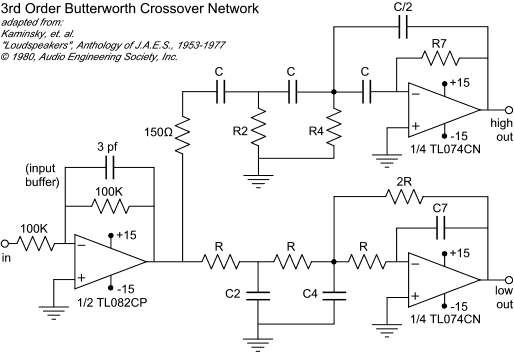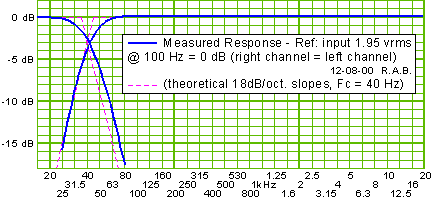## 電子式分頻器(濾波)線上計算器輸入想要的分頻頻率: Fol = Foh = Hz, 計算出的值直接配合上圖配置
R = C = µF
R2 = C2 = µF
R4 = C4 = µF
R7 = C7 = µF

R的理論值 ...
(頻率Fc < 200 Hz時選擇使用R = 100 KΩ，否則 R = 10 KΩ):
C = (10/R)*50
2 π F
C2 = 2455.3
2 π F R
C4 = 2108.9
2 π F R
C7 = 193.1
2 π F R

R2 = 407.4
2 π F C
R4 = 474.2
2 π F C
R7 = 5176.6
2 π F C
(C in

(R in
µF)

KΩ)